# Dirichlet series associated with holomorphic cusp forms

Level one modular forms. A cusp form of weight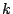for the full modular group is a holomorphic function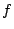on the upper half-plane which satisfies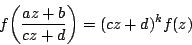for all integers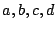with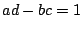and also has the property that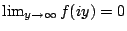. Cusp forms for the whole modular group exist only for even integers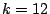and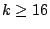. The cusp forms of a given weightof this form make a complex vector space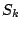of dimension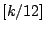if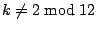and of dimension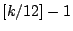if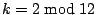. Each such vector space has a special basis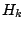of Hecke eigenforms which consist of functions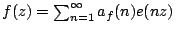for which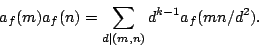The Fourier coefficients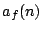are real algebraic integers of degree equal to the dimension of the vector space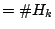. Thus, when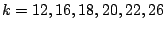the spaces are one dimensional and the coefficients are ordinary integers. The L-function associated with a Hecke formof weightis given by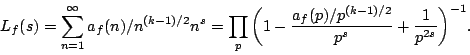By Deligne's theorem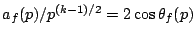for a real. It is conjectured (Sato-Tate) that for eachthe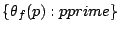is uniformly distributed on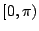with respect to the measure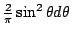. We write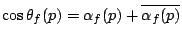where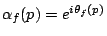; then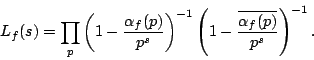The functional equation satisfied by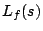is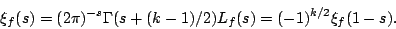Higher level forms. Let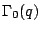denote the group of matrices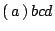with integerssatisfyingand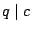. This group is called the Hecke congruence group. A functionholomorphic on the upper half plane satisfyingfor all matrices inandis called a cusp form for; the space of these is a finite dimensional vector space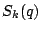. The spaceabove is the same as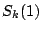. Again, these spaces are empty unlessis an even integer. Ifis an even integer, then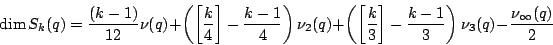where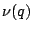is the index of the subgroupin the full modular group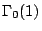: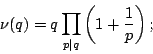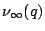is the number of cusps of: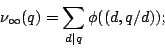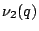is the number of inequivalent elliptic points of order 2:and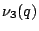is the number of inequivalent elliptic points of order 3:It is clear from this formula that the dimension ofgrows approximately linearly with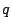and.

For the spacesthe issue of primitive forms and imprimitive forms arise, much as the situation with characters. In fact, one should think of the Fourier coefficients of cusp forms as being a generalization of characters. They are not periodic, but they act as harmonic detectors, much as characters do, through their orthogonality relations (below). Imprimitive cusp forms arise in two ways. Firstly , if, then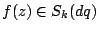for any integer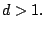Secondly, if, then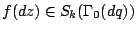for any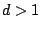. The dimension of the subspace of primitive forms is given by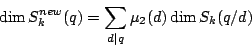where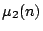is the multiplicative function defined for prime powers by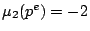if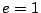,if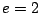, and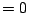if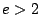. The subspace of newforms has a Hecke basis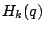consisting of primitive forms, or newforms, or Hecke forms. These can be identified as thosewhich have a Fourier series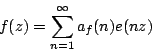where thehave the property that the associated L-function has an Euler product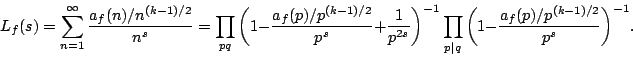The functional equation satisfied byis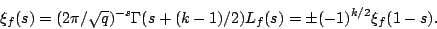Back to the main index for The Riemann Hypothesis.